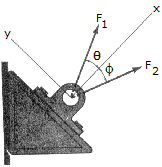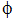# Engineering Mechanics - Force Vectors - Discussion

### Discussion :: Force Vectors - General Questions (Q.No.18)

18.If= 20° and= 35°, determine the magnitudes of F1 and F2 so that the resultant force has a magnitude of 20 lb and is directed along the positive x axis.

 [A]. F1 = 20.0 lb, F2 = 22.9 lb [B]. F1 = 25.6 lb, F2 = 26.6 lb [C]. F1 = 28.5 lb, F2 = 11.91 lb [D]. F1 = 14.00 lb, F2 = 8.35 lb

Explanation:

No answer description available for this question.

 Ismayil said: (Feb 15, 2017) Find obtuse angle of parallelogram (360-2*(20+35))/2 = 125. Sine law: 20/sin125 = F1/sin35 = F2/sin20. F1 = 14. F2 = 8.35.

 Sonm said: (Aug 6, 2017) Give More explanation.